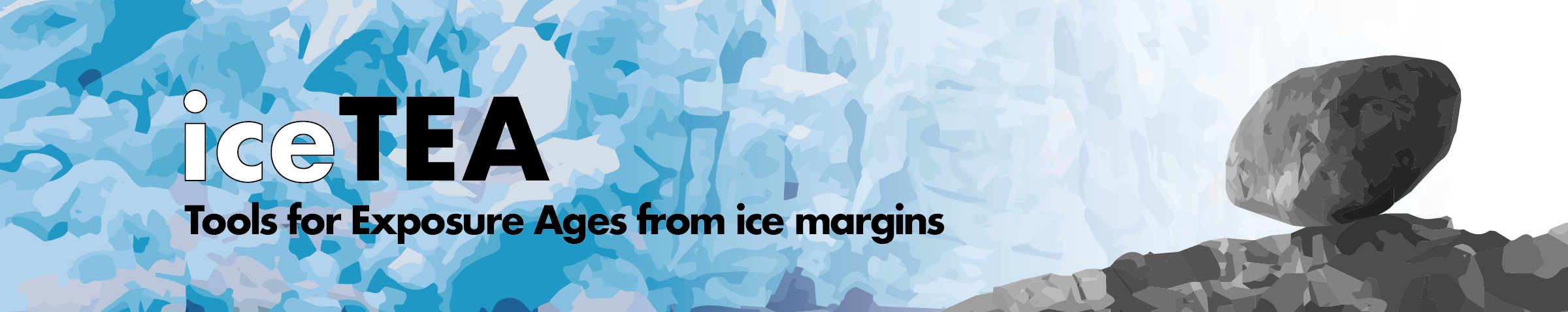## Correct for Elevation Change

The iceTEA tool determines past changes in sample elevation from either a glacial isostatic adjustment (GIA) model or a linear rate (uplift or subsidence), and then calculates exposure ages correcting for these changes. It uses a modified version of the CRONUScalc calculation framework (Marrero et al., 2016), with time-dependent elevation-latitude scaling factors, and global production rate calibration datasets (Borchers et al., 2016). The corrected ages can then be plotted as kernel density estimates.

Details are described here: Jones et al., 2019, Quaternary Geochronology (preprint version here).

Upload exposure age data in the ‘standard’ input form (as .xlsx, .csv or .txt), and choose preferred time-dependent production scaling model: ‘Lm’ (Lal, 1991); ‘LSD’ (Lifton et al., 2014); ‘LSDn’, nuclide-specific scaling (Lifton et al., 2014). Then select the elevation change method, either from a GIA model or as an average linear rate. NOTE: This is a calculation-intensive tool and may take 5 minutes per sample.